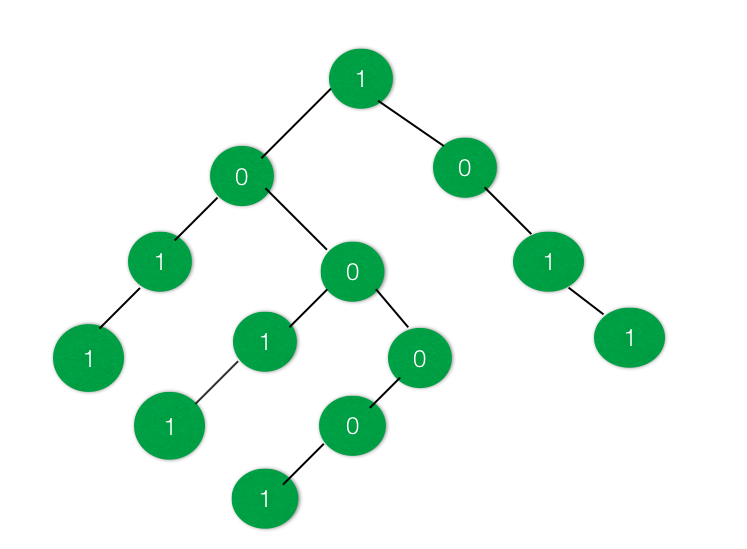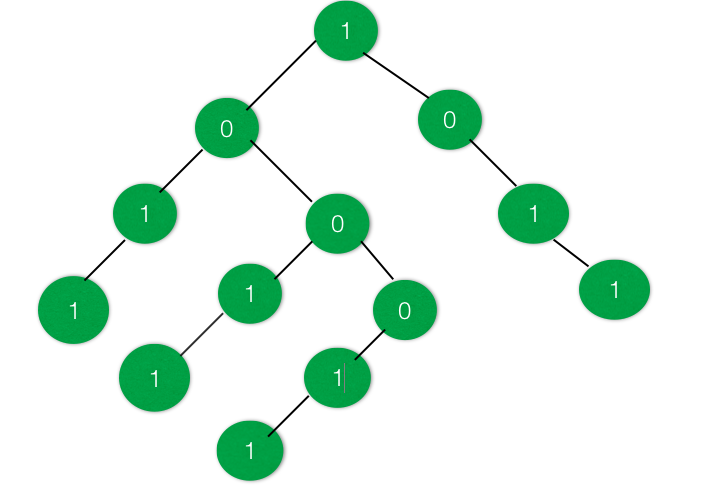# Check if the given Binary Tree have a Subtree with equal no of 1’s and 0’s

Given a Binary Tree having data at nodes as either 0’s or 1’s. The task is to find out whether there exists a subtree having an equal number of 1’s and 0’s.

Examples:

Input :Output : True
There are two subtrees present in the above tree where the number of 1’s is equal to the number of 0’s.

Input :Output : False
There is no such subtree present which has the number of 1’s equal to number of 0’s

Approach: The idea is to change the data 0’s of the tree to -1. So that it becomes very easy to find the subtree having equal number of 0’s and 1’s. After converting all 0’s to -1, create a sum tree. After creating the sum tree, each node will contain the sum of all node lying under it.

Traverse the tree again and find if there is a node having 0 sum, it means that there is a subtree that has the equal number of 1’s and -1’s, i.e. equal number of 1’s and 0’s.

Below is the implementation of the above approach:

## C++

 `// C++ program to check if there exist a ` `// subtree with equal number of 1's and 0's ` ` `  `#include ` `using` `namespace` `std; ` ` `  `// Binary Tree Node ` `struct` `node { ` `    ``int` `data; ` `    ``struct` `node *right, *left; ` `}; ` ` `  `// Utility function to create a new node ` `struct` `node* newnode(``int` `key) ` `{ ` `    ``struct` `node* temp = ``new` `node; ` `    ``temp->data = key; ` `    ``temp->right = NULL; ` `    ``temp->left = NULL; ` ` `  `    ``return` `temp; ` `} ` ` `  `// Function to convert all 0's in the ` `// tree to -1 ` `void` `convert(``struct` `node* root) ` `{ ` `    ``if` `(root == NULL) { ` `        ``return``; ` `    ``} ` ` `  `    ``// Move to right subtree ` `    ``convert(root->right); ` ` `  `    ``// Replace the 0's with -1 in the tree ` `    ``if` `(root->data == 0) { ` `        ``root->data = -1; ` `    ``} ` ` `  `    ``// Move to left subtree ` `    ``convert(root->left); ` `} ` ` `  `// Function to convert the tree to a SUM tree ` `int` `sum_tree(``struct` `node* root) ` `{ ` `    ``int` `a = 0, b = 0; ` ` `  `    ``if` `(root == NULL) { ` `        ``return` `0; ` `    ``} ` ` `  `    ``a = sum_tree(root->left); ` `    ``b = sum_tree(root->right); ` ` `  `    ``root->data = root->data + a + b; ` ` `  `    ``return` `root->data; ` `} ` ` `  `// Function to check if there exists a subtree ` `// with equal no of 1s and 0s ` `int` `checkSubtree(``struct` `node* root, ``int` `d) ` `{ ` `    ``if` `(root == NULL) { ` `        ``return` `0; ` `    ``} ` ` `  `    ``// Check if there is a subtree with equal ` `    ``// 1s and 0s or not ` `    ``if` `(d == 0) { ` `        ``d = checkSubtree(root->left, d); ` `    ``} ` ` `  `    ``if` `(root->data == 0) { ` `        ``d = 1; ` `        ``return` `d; ` `    ``} ` ` `  `    ``if` `(d == 0) { ` `        ``d = checkSubtree(root->right, d); ` `    ``} ` ` `  `    ``return` `d; ` `} ` ` `  `// Driver Code ` `int` `main() ` `{ ` `    ``// Create the Binary Tree ` `    ``struct` `node* root = newnode(1); ` `    ``root->right = newnode(0); ` `    ``root->right->right = newnode(1); ` `    ``root->right->right->right = newnode(1); ` `    ``root->left = newnode(0); ` `    ``root->left->left = newnode(1); ` `    ``root->left->left->left = newnode(1); ` `    ``root->left->right = newnode(0); ` `    ``root->left->right->left = newnode(1); ` `    ``root->left->right->left->left = newnode(1); ` `    ``root->left->right->right = newnode(0); ` `    ``root->left->right->right->left = newnode(0); ` `    ``root->left->right->right->left->left = newnode(1); ` ` `  `    ``// Convert all 0s in tree to -1 ` `    ``convert(root); ` ` `  `    ``// Convert the tree into a SUM tree ` `    ``sum_tree(root); ` ` `  `    ``// Check if required Subtree exists ` `    ``int` `d = 0; ` `    ``if` `(checkSubtree(root, d)) { ` `        ``cout << ``"True"` `<< endl; ` `    ``} ` `    ``else` `{ ` `        ``cout << ``"False"` `<< endl; ` `    ``} ` ` `  `    ``return` `0; ` `} `

## Java

 `// Java program to check if there exist a ` `// subtree with equal number of 1's and 0's ` ` `  `import` `java.util.*; ` `class` `GFG{ ` ` `  `    ``// Binary Tree Node ` `    ``static` `class` `node { ` `        ``int` `data; ` `        ``node right, left; ` `    ``}; ` `     `  `    ``// Utility function to create a new node ` `    ``static` `node newnode(``int` `key) ` `    ``{ ` `        ``node temp = ``new` `node(); ` `        ``temp.data = key; ` `        ``temp.right = ``null``; ` `        ``temp.left = ``null``; ` `     `  `        ``return` `temp; ` `    ``} ` `     `  `    ``// Function to convert all 0's in the ` `    ``// tree to -1 ` `    ``static` `void` `convert(node root) ` `    ``{ ` `        ``if` `(root == ``null``) { ` `            ``return``; ` `        ``} ` `     `  `        ``// Move to right subtree ` `        ``convert(root.right); ` `     `  `        ``// Replace the 0's with -1 in the tree ` `        ``if` `(root.data == ``0``) { ` `            ``root.data = -``1``; ` `        ``} ` `     `  `        ``// Move to left subtree ` `        ``convert(root.left); ` `    ``} ` `     `  `    ``// Function to convert the tree to a SUM tree ` `    ``static` `int` `sum_tree(node root) ` `    ``{ ` `        ``int` `a = ``0``, b = ``0``; ` `     `  `        ``if` `(root == ``null``) { ` `            ``return` `0``; ` `        ``} ` `     `  `        ``a = sum_tree(root.left); ` `        ``b = sum_tree(root.right); ` `     `  `        ``root.data = root.data + a + b; ` `     `  `        ``return` `root.data; ` `    ``} ` `     `  `    ``// Function to check if there exists a subtree ` `    ``// with equal no of 1s and 0s ` `    ``static` `int` `checkSubtree(node root, ``int` `d) ` `    ``{ ` `        ``if` `(root == ``null``) { ` `            ``return` `0``; ` `        ``} ` `     `  `        ``// Check if there is a subtree with equal ` `        ``// 1s and 0s or not ` `        ``if` `(d == ``0``) { ` `            ``d = checkSubtree(root.left, d); ` `        ``} ` `     `  `        ``if` `(root.data == ``0``) { ` `            ``d = ``1``; ` `            ``return` `d; ` `        ``} ` `     `  `        ``if` `(d == ``0``) { ` `            ``d = checkSubtree(root.right, d); ` `        ``} ` `     `  `        ``return` `d; ` `    ``} ` `     `  `    ``// Driver Code ` `    ``public` `static` `void` `main(String args[]) ` `    ``{ ` `        ``// Create the Binary Tree ` `        ``node root = newnode(``1``); ` `        ``root.right = newnode(``0``); ` `        ``root.right.right = newnode(``1``); ` `        ``root.right.right.right = newnode(``1``); ` `        ``root.left = newnode(``0``); ` `        ``root.left.left = newnode(``1``); ` `        ``root.left.left.left = newnode(``1``); ` `        ``root.left.right = newnode(``0``); ` `        ``root.left.right.left = newnode(``1``); ` `        ``root.left.right.left.left = newnode(``1``); ` `        ``root.left.right.right = newnode(``0``); ` `        ``root.left.right.right.left = newnode(``0``); ` `        ``root.left.right.right.left.left = newnode(``1``); ` `     `  `        ``// Convert all 0s in tree to -1 ` `        ``convert(root); ` `     `  `        ``// Convert the tree into a SUM tree ` `        ``sum_tree(root); ` `     `  `        ``// Check if required Subtree exists ` `        ``int` `d = ``0``; ` `        ``if` `(checkSubtree(root, d)>=``1``) { ` `            ``System.out.println(``"True"``); ` `        ``} ` `        ``else` `{ ` `            ``System.out.println(``"False"``); ` `        ``} ` `    ``} ` `} ` ` `  ` `  `// This code is contributed by AbhiThakur `

## C#

 `// C# program to check if there exist a ` `// subtree with equal number of 1's and 0's ` `using` `System; ` ` `  `class` `GFG{ ` `  `  `    ``// Binary Tree Node ` `    ``class` `node { ` `        ``public` `int` `data; ` `        ``public` `node right, left; ` `    ``}; ` `      `  `    ``// Utility function to create a new node ` `    ``static` `node newnode(``int` `key) ` `    ``{ ` `        ``node temp = ``new` `node(); ` `        ``temp.data = key; ` `        ``temp.right = ``null``; ` `        ``temp.left = ``null``; ` `      `  `        ``return` `temp; ` `    ``} ` `      `  `    ``// Function to convert all 0's in the ` `    ``// tree to -1 ` `    ``static` `void` `convert(node root) ` `    ``{ ` `        ``if` `(root == ``null``) { ` `            ``return``; ` `        ``} ` `      `  `        ``// Move to right subtree ` `        ``convert(root.right); ` `      `  `        ``// Replace the 0's with -1 in the tree ` `        ``if` `(root.data == 0) { ` `            ``root.data = -1; ` `        ``} ` `      `  `        ``// Move to left subtree ` `        ``convert(root.left); ` `    ``} ` `      `  `    ``// Function to convert the tree to a SUM tree ` `    ``static` `int` `sum_tree(node root) ` `    ``{ ` `        ``int` `a = 0, b = 0; ` `      `  `        ``if` `(root == ``null``) { ` `            ``return` `0; ` `        ``} ` `      `  `        ``a = sum_tree(root.left); ` `        ``b = sum_tree(root.right); ` `      `  `        ``root.data = root.data + a + b; ` `      `  `        ``return` `root.data; ` `    ``} ` `      `  `    ``// Function to check if there exists a subtree ` `    ``// with equal no of 1s and 0s ` `    ``static` `int` `checkSubtree(node root, ``int` `d) ` `    ``{ ` `        ``if` `(root == ``null``) { ` `            ``return` `0; ` `        ``} ` `      `  `        ``// Check if there is a subtree with equal ` `        ``// 1s and 0s or not ` `        ``if` `(d == 0) { ` `            ``d = checkSubtree(root.left, d); ` `        ``} ` `      `  `        ``if` `(root.data == 0) { ` `            ``d = 1; ` `            ``return` `d; ` `        ``} ` `      `  `        ``if` `(d == 0) { ` `            ``d = checkSubtree(root.right, d); ` `        ``} ` `      `  `        ``return` `d; ` `    ``} ` `      `  `    ``// Driver Code ` `    ``public` `static` `void` `Main(String []args) ` `    ``{ ` `        ``// Create the Binary Tree ` `        ``node root = newnode(1); ` `        ``root.right = newnode(0); ` `        ``root.right.right = newnode(1); ` `        ``root.right.right.right = newnode(1); ` `        ``root.left = newnode(0); ` `        ``root.left.left = newnode(1); ` `        ``root.left.left.left = newnode(1); ` `        ``root.left.right = newnode(0); ` `        ``root.left.right.left = newnode(1); ` `        ``root.left.right.left.left = newnode(1); ` `        ``root.left.right.right = newnode(0); ` `        ``root.left.right.right.left = newnode(0); ` `        ``root.left.right.right.left.left = newnode(1); ` `      `  `        ``// Convert all 0s in tree to -1 ` `        ``convert(root); ` `      `  `        ``// Convert the tree into a SUM tree ` `        ``sum_tree(root); ` `      `  `        ``// Check if required Subtree exists ` `        ``int` `d = 0; ` `        ``if` `(checkSubtree(root, d) >= 1) { ` `            ``Console.WriteLine(``"True"``); ` `        ``} ` `        ``else` `{ ` `            ``Console.WriteLine(``"False"``); ` `        ``} ` `    ``} ` `} ` ` `  `// This code is contributed by sapnasingh4991 `

Output:

```True
```

Time Complexity: O(N)
Space Complexity: O(1)

GeeksforGeeks has prepared a complete interview preparation course with premium videos, theory, practice problems, TA support and many more features. Please refer Placement 100 for details

My Personal Notes arrow_drop_upCheck out this Author's contributed articles.

If you like GeeksforGeeks and would like to contribute, you can also write an article using contribute.geeksforgeeks.org or mail your article to contribute@geeksforgeeks.org. See your article appearing on the GeeksforGeeks main page and help other Geeks.

Please Improve this article if you find anything incorrect by clicking on the "Improve Article" button below.